Problems on Curve Fitting:

Example-1 Fit straight line for the following data: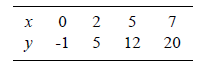Solutions: Let straight line is a bx. Normal equation for straight lines are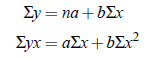Form the following table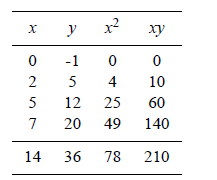Now normal equations reduces to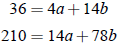On solving,  a = −1.1381 and b = 2.8966

Hence the fitted straight line is

y = −1.1381 2.8966x

Example-2 Fit a straight line to the following data :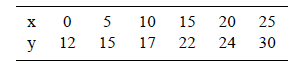Solutions: Here n = 6, i.e., even and the values of x are equally spaced , h is equal to 5. Therefore, to make the calculation easier, we take the unit of measurement h/2 , i.e., 2.5 and the origin at the mean of two middle terms 10 and 15, i.e., origin is taken at 1/2 (10 15) = 12.5.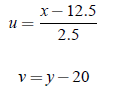Let the equation of straight line be

v = a bu

Then the normal equation are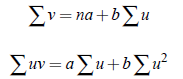So,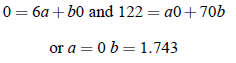Thus, the required equation of straight line is

y = 0.7x 11.285